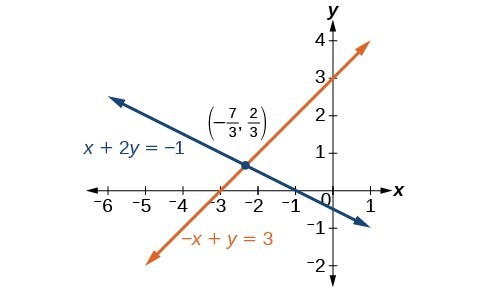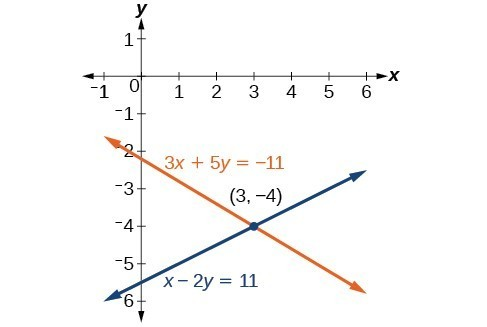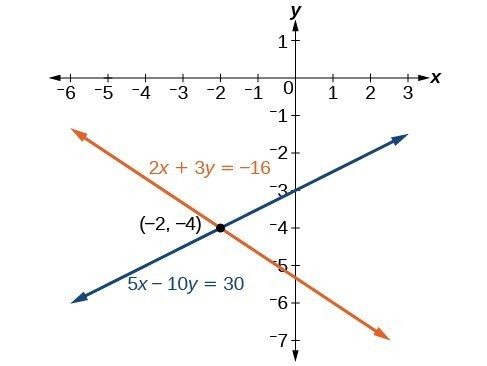Solving Systems of Equations in Two Variables by the Addition Method

A third method of solving systems of linear equations is the addition method. In this method, we add two terms with the same variable, but opposite coefficients, so that the sum is zero. Of course, not all systems are set up with the two terms of one variable having opposite coefficients. Often we must adjust one or both of the equations by multiplication so that one variable will be eliminated by addition.

How To: Given a system of equations, solve using the addition method.

1. Write both equations with x– and y-variables on the left side of the equal sign and constants on the right.
2. Write one equation above the other, lining up corresponding variables. If one of the variables in the top equation has the opposite coefficient of the same variable in the bottom equation, add the equations together, eliminating one variable. If not, use multiplication by a nonzero number so that one of the variables in the top equation has the opposite coefficient of the same variable in the bottom equation, then add the equations to eliminate the variable.
3. Solve the resulting equation for the remaining variable.
4. Substitute that value into one of the original equations and solve for the second variable.
5. Check the solution by substituting the values into the other equation.

Example 4: Solving a System by the Addition Method

Solve the given system of equations by addition.

$\begin{array}{l}x+2y=-1\hfill \\ -x+y=3\hfill \end{array}$

Solution

Both equations are already set equal to a constant. Notice that the coefficient of $x$ in the second equation, –1, is the opposite of the coefficient of $x$ in the first equation, 1. We can add the two equations to eliminate $x$ without needing to multiply by a constant.

$\frac{\begin{array}{l}\hfill \\ x+2y=-1\hfill \\ -x+y=3\hfill \end{array}}{\text{}\text{}\text{}\text{}\text{}3y=2}$

Now that we have eliminated $x$, we can solve the resulting equation for $y$.

$\begin{array}{l}3y=2\hfill \\ \text{ }y=\frac{2}{3}\hfill \end{array}$

Then, we substitute this value for $y$ into one of the original equations and solve for $x$.

$\begin{array}{l}\text{ }-x+y=3\hfill \\ \text{ }-x+\frac{2}{3}=3\hfill \\ \text{ }-x=3-\frac{2}{3}\hfill \\ \text{ }-x=\frac{7}{3}\hfill \\ \text{ }x=-\frac{7}{3}\hfill \end{array}$

The solution to this system is $\left(-\frac{7}{3},\frac{2}{3}\right)$.

Check the solution in the first equation.

$\begin{array}{llll}\text{ }x+2y=-1\hfill & \hfill & \hfill & \hfill \\ \text{ }\left(-\frac{7}{3}\right)+2\left(\frac{2}{3}\right)=\hfill & \hfill & \hfill & \hfill \\ \text{ }-\frac{7}{3}+\frac{4}{3}=\hfill & \hfill & \hfill & \hfill \\ \text{ }-\frac{3}{3}=\hfill & \hfill & \hfill & \hfill \\ \text{ }-1=-1\hfill & \hfill & \hfill & \text{True}\hfill \end{array}$

Analysis of the Solution

We gain an important perspective on systems of equations by looking at the graphical representation. See Figure 5 to find that the equations intersect at the solution. We do not need to ask whether there may be a second solution because observing the graph confirms that the system has exactly one solution.Figure 5

Example 5: Using the Addition Method When Multiplication of One Equation Is Required

Solve the given system of equations by the addition method.

$\begin{array}{l}3x+5y=-11\hfill \\ \hfill \\ x - 2y=11\hfill \end{array}$

Solution

Adding these equations as presented will not eliminate a variable. However, we see that the first equation has $3x$ in it and the second equation has $x$. So if we multiply the second equation by $-3,\text{}$ the x-terms will add to zero.

$\begin{array}{llll}\text{ }x - 2y=11\hfill & \hfill & \hfill & \hfill \\ -3\left(x - 2y\right)=-3\left(11\right)\hfill & \hfill & \hfill & \text{Multiply both sides by }-3.\hfill \\ \text{ }-3x+6y=-33\hfill & \hfill & \hfill & \text{Use the distributive property}.\hfill \end{array}$

$\begin{array}\ \hfill 3x+5y=−11 \\ \hfill −3x+6y=−33 \\ \text{_____________} \\ \hfill 11y=−44 \\ \hfill y=−4 \end{array}$

For the last step, we substitute $y=-4$ into one of the original equations and solve for $x$.

$\begin{array}{c}3x+5y=-11\\ 3x+5\left(-4\right)=-11\\ 3x - 20=-11\\ 3x=9\\ x=3\end{array}$

Our solution is the ordered pair $\left(3,-4\right)$. Check the solution in the original second equation.

$\begin{array}{llll}\text{ }x - 2y=11\hfill & \hfill & \hfill & \hfill \\ \left(3\right)-2\left(-4\right)=3+8\hfill & \hfill & \hfill & \hfill \\ \text{ }=11\hfill & \hfill & \hfill & \text{True}\hfill \end{array}$Figure 6

Try It 4

Solve the system of equations by addition.

$\begin{array}{c}2x - 7y=2\\ 3x+y=-20\end{array}$

Solution

Example 6: Using the Addition Method When Multiplication of Both Equations Is Required

Solve the given system of equations in two variables by addition.

$\begin{array}{c}2x+3y=-16\\ 5x - 10y=30\end{array}$

Solution

One equation has $2x$ and the other has $5x$. The least common multiple is $10x$ so we will have to multiply both equations by a constant in order to eliminate one variable. Let’s eliminate $x$ by multiplying the first equation by $-5$ and the second equation by $2$.

$\begin{array}{l} -5\left(2x+3y\right)=-5\left(-16\right)\hfill \\ \text{ }-10x - 15y=80\hfill \\ \text{ }2\left(5x - 10y\right)=2\left(30\right)\hfill \\ \text{ }10x - 20y=60\hfill \end{array}$

Then, we add the two equations together.

$\begin{array}\ −10x−15y=80 \\ 10x−20y=60 \\ \text{______________} \\ \text{ }−35y=140 \\ y=−4 \end{array}$

Substitute $y=-4$ into the original first equation.

$\begin{array}{c}2x+3\left(-4\right)=-16\\ 2x - 12=-16\\ 2x=-4\\ x=-2\end{array}$

The solution is $\left(-2,-4\right)$. Check it in the other equation.

$\begin{array}{r}\hfill \text{ }5x - 10y=30\\ \hfill 5\left(-2\right)-10\left(-4\right)=30\\ \hfill \text{ }-10+40=30\\ \hfill \text{ }30=30\end{array}$Figure 7

Example 7: Using the Addition Method in Systems of Equations Containing Fractions

Solve the given system of equations in two variables by addition.

$\begin{array}{l}\frac{x}{3}+\frac{y}{6}=3\hfill \\ \frac{x}{2}-\frac{y}{4}=\text{ }1\hfill \end{array}$

Solution

First clear each equation of fractions by multiplying both sides of the equation by the least common denominator.

$\begin{array}{l}6\left(\frac{x}{3}+\frac{y}{6}\right)=6\left(3\right)\hfill \\ \text{ }2x+y=18\hfill \\ 4\left(\frac{x}{2}-\frac{y}{4}\right)=4\left(1\right)\hfill \\ \text{ }2x-y=4\hfill \end{array}$

Now multiply the second equation by $-1$ so that we can eliminate the x-variable.

$\begin{array}{l}-1\left(2x-y\right)=-1\left(4\right)\hfill \\ \text{ }-2x+y=-4\hfill \end{array}$

Add the two equations to eliminate the x-variable and solve the resulting equation.

$\begin{array}\ \hfill 2x+y=18 \\ \hfill−2x+y=−4 \\ \text{_____________} \\ \hfill 2y=14 \\ \hfill y=7 \end{array}$

Substitute $y=7$ into the first equation.

$\begin{array}{l}2x+\left(7\right)=18\hfill \\ \text{ }2x=11\hfill \\ \text{ }x=\frac{11}{2}\hfill \\ \text{ }=7.5\hfill \end{array}$

The solution is $\left(\frac{11}{2},7\right)$. Check it in the other equation.

$\begin{array}{c}\frac{x}{2}-\frac{y}{4}=1\\ \frac{\frac{11}{2}}{2}-\frac{7}{4}=1\\ \frac{11}{4}-\frac{7}{4}=1\\ \frac{4}{4}=1\end{array}$

Try It 5

Solve the system of equations by addition.

$\begin{array}{c}2x+3y=8\\ 3x+5y=10\end{array}$

Solution